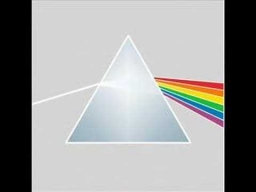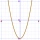Please determine the solvability conditions of the equation, solve the equation and perform the test:

x divided by x squared minus 2x plus1 the whole minus x + 3 divided by x squared minus 1 this is equal to 0:

x/(x2-2x+1) - (x+3)/( x2-1) = 0

Correct result:

x =  3

#### Solution:

Our quadratic equation calculator calculates it.We would be pleased if you find an error in the word problem, spelling mistakes, or inaccuracies and send it to us. Thank you!Tips to related online calculators
Looking for help with calculating roots of a quadratic equation?
Need help calculate sum, simplify or multiply fractions? Try our fraction calculator.
Do you have a linear equation or system of equations and looking for its solution? Or do you have quadratic equation?

## Next similar math problems:

• Eq2 2Solve following equation with quadratic members and rational function: (x2+1)/(x-4) + (x2-1)/(x+3) = 23
• VCP equationSolve the following equation with variations, combinations and permutations: 4 V(2,x)-3 C(2,x+ 1) - x P(2) = 0
• ProveProve that k1 and k2 is the equations of two circles. Find the equation of the line that passes through the centers of these circles. k1: x2+y2+2x+4y+1=0 k2: x2+y2-8x+6y+9=0
• Intersections 3Find the intersections of the circles x2 + y2 + 6 x - 10 y + 9 = 0 and x2 + y2 + 18 x + 4 y + 21 = 0
• Domains of functionsF(x)=x2-7x and g(x)=5-x2 Domain of (fg)(x) is. .. . . Domain of (f/g)(x). ..
• Simple equation 6Solve equation with one variable: X/2+X/3+X/4=X+4Find the roots of the quadratic equation: 3x2-4x + (-4) = 0.
• Linear systemSolve a set of two equations of two unknowns: 1.5x+1.2y=0.6 0.8x-0.2y=2
• Find the 20Find the product and the sum of the roots of x2 + 3x - 9 = 0
• Sphere equationObtain the equation of sphere its centre on the line 3x+2z=0=4x-5y and passes through the points (0,-2,-4) and (2,-1,1).
• TrapeziumThe lengths of a parallel sides of a trapezium are (2x+3) and (x+8) and the distance between them is (x+4). if the area of the trapezium is 590 , find the value of x.
• Curve and lineThe equation of a curve C is y=2x² -8x+9 and the equation of a line L is x+ y=3 (1) Find the x co-ordinates of the points of intersection of L and C. (2) Show that one of these points is also the stationary point of C?Which of the points belong function f:y= 2x2- 3x + 1 : A(-2, 15) B (3,10) C (1,4)If the square root of 3m2 +22 and -x = 0, and x=7, what is m?Determine the distance of a point B[1, -3] from the perpendicular projection of a point A[3, -2] on a straight line 2 x + y + 1 = 0.Equation of sphere with three point (a,0,0), (0, a,0), (0,0, a) and center lies on plane x+y+z=aSolve quadratic equation: 2x2-58x+396=0